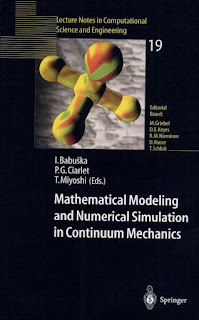APPLIED MATHEMATICS

# Mathematical Modeling and Numerical Simulation in Continuum Mechanics PDF Free DownloadThis book shows the latest frontiers of the research by the most active researchers in the field of numerical mathematics. The papers in the book were presented in a symposium at Yamaguchi, Japan. The subject of the symposium was mathematical modeling and numerical simulation in continuum mechanics. The topics of the lectures ranged from solids to fluids and included both mathematical and computational analysis of phenomena and algorithms. The readers can study the latest results on shells, plates, flows in various situations, fracture of solids, new ways of exact error estimates and many other topics.